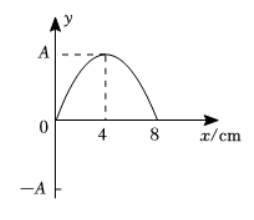$\text{A.}$ 该波的频率为 $100 \mathrm{~Hz}$, 波速为 $8.0 \mathrm{~m} / \mathrm{s}$ $\text{B.}$ $x=24 \mathrm{~cm}$ 处的质点在 $0.025 \mathrm{~s}$ 时第一次到达波峰的位置 $\text{C.}$ $x=32 \mathrm{~cm}$ 处的质点在 $\frac{13}{300} s$ 时第一次到达 $\frac{1}{2} A$ 的位置 $\text{D.}$ $x=48 \mathrm{~cm}$ 处的质点在 $\frac{1}{16} s$ 时第一次到达 $\frac{\sqrt{2}}{2} A$ 的位置

D

#### 解析：

$B$ 、波的传播时波形向前平移, $0.01 \mathrm{~s}$ 时波峰在 $x=4 \mathrm{~cm}$ 处, 当 $t=0.01 \mathrm{~s}$ 时 $x=4 \mathrm{~cm}$ 处的波峰第一次传到 $x=24 \mathrm{~cm}$ 处时, $x=2$
$4 \mathrm{~cm}$ 处的质点第一次到达波峰, 则 $x=24 \mathrm{~cm}$ 处的质点第一次到达波峰的位置的时刻为 $t_0=\frac{x_0}{v}=\frac{(24-4) \times 10^{-2}}{8} s+0.01 \mathrm{~s}=0$.
$035 s$,故 $B$ 错误；
$C$ 、由图可知, 该波的起振方向沿 $y$ 轴正方向, 波源的振动方程为 $y=A \sin \left(\frac{2 \pi}{T} t\right)$ 。

$D$ 、波传播到 $x=48 \mathrm{~cm}$ 处的时间 $t_3=\frac{x_3}{v}=\frac{48 \times 10^{-2}}{8} s=0.06 \mathrm{~s}, x=48 \mathrm{~cm}$ 处的质点从起振到第一次到达 $\frac{\sqrt{2}}{2} A$ 位置的时间 $\frac{\sqrt{2}}{2} A=\sin \left(\frac{2 \pi}{T} t_4\right)$